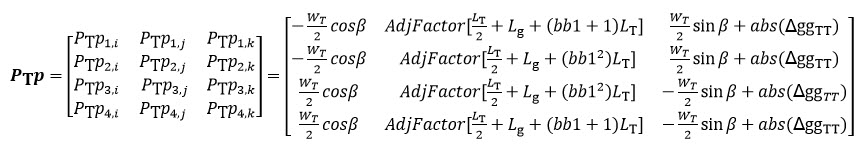This series of functions computes the shading factors on the direct beam component of irradiance. Given the sun’s azimuth angle and zenith angle (elevation angle’s complement), as well as the array’s orientation, spacing, tilt, and dimensions, the functions compute the geometry of the shadow cast by one row on an adjacent row as well as the fraction of the array that is shaded by tables within the array. Edge effects can be excluded based on user selection, which represents ignoring the unshaded edges of rows for DC fields that do not represent full arrays, typically used for the case when the array is designed for string inverters.

Figure 10 illustrates the direct beam row-to-row shading. The current tilt of the modules is represented here by 𝛽 for the fixed tilt or tracker tilt angle.### Figure 10. One-dimensional shed shading illustration of either a fixed-tilt or tracker, as viewed from the edge of the row

The following diagram, Figure 11, illustrates the variables used to calculate the direct beam shading on an array. In the figure, Δpp is the distance between tables, LT is the length of each table, and WT is the width of each table. Nr is the number of sheds. Although not shown, module azimuth angle, γ, is defined with due north being 0°, and is dependent upon user input DC field orientation. Also not shown, NT is the number of tables per shed. Although Figure 11 illustrates a fixed tilt array, all geometric definitions are the same for horizontal tracking arrays.### Figure 11. Two-dimensional mutual shed-on-shed shading. Example shows a fixed-tilt array with 180 azimuth (south-facing). Diagram adapted from: Dan Weinstock, Joseph Appelbaum, Shadow Variation on Photovoltaic Collectors in a Solar Field. Proceedings of the 23rd IEEE Convention of Electrical and Electronics Engineers in Israel, 2004.

Figure 12 shows the geometries that define a terrain slope. The angle of the slope is defined as 𝛼. This can be measured as the angle between the vector normal to the surface of the terrain and the zenith. The direction faced by the slope, called the aspect, is the azimuth from north of the vector normal to the surface of the terrain, γg.### Figure 12. Geometry of a slope. Terrain slope 𝛼 and aspect γg. Figure adapted and recreated from http://www.ces.iisc.ernet.in/grass/grass72/manuals/i.topo.corr.html

The diagram in Figure 13 illustrates the additional variables of slope 𝛼 and aspect γg used for modeling an array that is built along a slope. The module azimuth of the array points south, but the slope geometry is independent of the module azimuth.## InputsThe incidence angle is not used to compute the shading factor per se, but is a test to verify if the sun is behind the plane of the array.

## Outputs## Algorithm (Vector Method)

### Decomposition of a Slope

The terrain slope angle 𝛼 can be defined by the user for any aspect, or azimuth direction, γg. The aspect is defined according to the same convention as module azimuth angle.

To evaluate the shading that results from such a slope, it is helpful to decompose the slope into the two separate slopes that are along and perpendicular to the azimuth angle of the modules.

The component of the slope that is along the module azimuth angle affects the shading that occurs from one row onto another, or row-to-row shading. Since this shading occurs across rows, it is being termed Transverse shading. The contours of the slope in the transverse direction run parallel to the rows.

The component of the slope perpendicular to the module azimuth angle is along the rows of the array. It causes the tables of the rows to be at staggered heights, for a horizontal tracker system. This slope therefore creates shading between neighboring tables along a row, which is being termed Adjacent shading, or table-to-table shading. The overall shading impact of the slope 𝛼 is the shading due to both components. Depending on the relative location of a given table in the array, it might cast either Transverse shading, Adjacent shading, or a combination of the two.

The component of the slope that affects the Transverse shading between neighboring rows at a positive tilt is defined as transverse slope, 𝜓. Transverse shading is illustrated in Figure 14.

The component of the slope that affects the Adjacent shading between neighboring tables is defined as adjacent slope, 𝜙. For a tracker system, an adjacent slope facing away from the equator is considered positive. For a fixed tilt system, a westward facing adjacent slope is considered positive. Figure 15 illustrates the adjacent shading created between tables within a row at different heights along the slope.### Figure 14. Treatment of sheds on terrain with a transverse slope, 𝜓, showing the horizontal post-to-post spacing, Δpp. The figure could be interpreted as either: (a) the edge-view (looking east) of a northern-hemisphere fixed-tilt array on a north-facing slope at noon (sun due south at right), or alternatively: (b) looking north at a horizontal tracker on a west-facing slope at sunrise.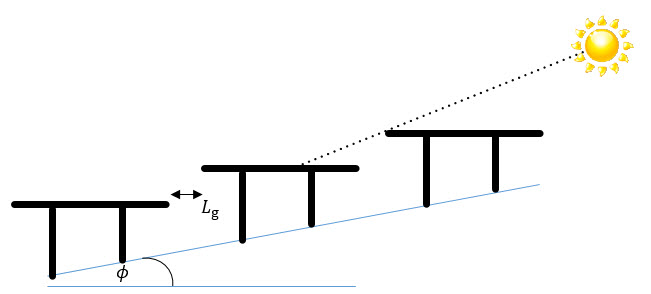### Figure 15. Treatment of sheds on terrain with an adjacent slope, 𝜙, showing the horizontal table-to-table spacing Lg of the slope angle 𝜓. The figure could be interpreted as either: (a) the front-view (looking east) of a northern-hemisphere horizontal tracker row on a north-facing slope at noon (sun due south at right), or alternatively: (b) looking north at a fixed tilt row on an west-facing slope at sunrise.

The components of the slope are decomposed into the perpendicular directions with the following calculations. The ground slope α can be positive or negative, while the aspect γg must be positive, from 0 to 2𝜋.

For a fixed tilt system with a positive latitude:For a fixed tilt system with a negative latitude or a tracker system with a positive latitude:For a tracker system with a negative latitude:The generalized shading fraction is calculated in the following algorithm.

1. First, define a right-handed table-centric Cartesian coordinate system for a 2-row geometry:

Origin:    Center of second table, at axis of rotation.

i:              positive in the direction of the first table (i.e. positive in the south direction for south-facing arrays).

j:              positive to the right along the lengthwise centerline of second table (from perspective of observer facing table).

k:             positive in the direction of the zenith.

The table causing shading in the row-to-row direction or the table-to-table direction (lengthwise along the rows) is the primary table, PTp or Prp, respectively, or Pp in general. The table that is shaded is the secondary table, Sp.Figure 16. Primary tables Pp relative to the shaded table Sp at a time other than solar noon. Primary table across the rows (or within the column), Prp, which creates Transverse shading. Primary table along (within) the row, PTp , which causes Adjacent (table-to-table) shading. The figure could be interpreted as either: (a) a N-S axis tracker system, or alternatively: (b) a south-facing fixed tilt system.

2. The solar unit vector s, transformed from spherical to the table-centric Cartesian coordinates, is:3. To calculate the shadow, we need to determine the vertical spacing between a corresponding point on the primary table (Pp) and the shaded table (Sp), conveniently determined by the height difference at the tops of corresponding posts of the tables. This spacing is defined as the glass-to-glass height difference Δgg.

4. The general equation of a plane is defined as a*x + b*y + c*z = 0. In our case, the tilted PV plane Sp intersects the plane normal to the surface of the earth at the y axis of our coordinate system, so the second row’s table sits in the plane a*x + c*z = 0, where a = sin(𝛽) and c = cos(𝛽). The equation for the plane in which the secondary table lies is therefore: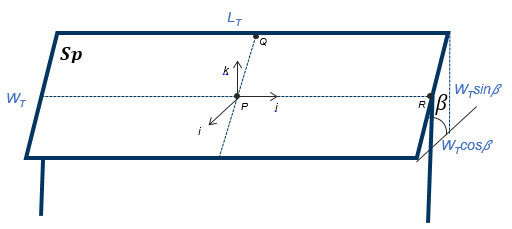### Figure 17. Detailed shaded table  geometry and coordinate system.

5. To find the percentage shaded as a function of the solar unit vector, first define the four vertices (the four corners) of the primary (Pp) table.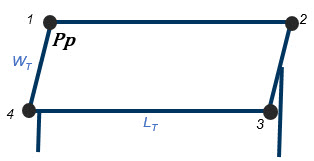### Figure 18. Vertex assignments of primary (Pp) table

The i,j,k coordinates of these vertices are given in the following matrix. The vertices are listed in the rows of the matrix, starting on the top-left of the table, and proceeding clockwise.6. Find the projection of the solar unit vector at each of these points Pp onto the plane containing the secondary table amounts to findthe intersection of the plane containing the secondary table with solar rays grazing each vertex of the primary table.### Figure 19. The projection of each of the points Prp along the solar unit vector s onto the plane containing the secondary table, where r is distance the solar ray has to travel between the two planes. This is at a time other than solar noon.

This can be evaluated as follows. The parameterization of the line made up of the solar ray passing through each vertex, n, as a function of r is given by:Here s is the solar unit vector. Substitute the formula for the line into the equation of the plane:Here r has become the distance the solar ray has to travel between the two tables. Determine the value of r, where a = sin 𝛽 and b = cos 𝛽: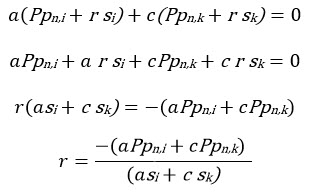Substitute r into the formula for the line to find the point of intersection in the secondary plane of the solar rays through each of the primary table’s vertices.7. Project the shading points Sp from 3D to 2D space, where y runs along the length of the table and x runs along the width of the table, as shown in Figure 20.### Figure 20. Relative locations of the shadow vertices P (shadow greyed) on the shaded table in 2D space.

In this coordinate system, y is simply the y-coordinate in the 3D panel-centric coordinate system, plus half the table length; x is the hypotenuse of i and k, added or subtracted from half the table width depending on the direction of i relative to the center of the table:8. If only one shadow falls on the table, it would be sufficient to determine the location of the corner of the shadow within the table to calculate the portion of the table that is shaded. However, if there might be multiple shadows on the table, the location of each shadow must be determined.

Next, find the location of the corners of PTn, the rectangular subset of the shadow that falls within the secondary table. The x-component of the shadow that exists on the secondary table is determined by testing the x-component of the first and third vertices of the shadow P against the x-component of the first and third vertices of the secondary table. The distance y is similarly determined with the same vertices. PTn can be calculated using either pair of opposite corners. It is assumed the planes of the tables are parallel to each other. The 2-dimensional coordinates of the secondary table’s vertices are given by V.9. The linear shading fraction SF of a single shadow on one table is then found by2: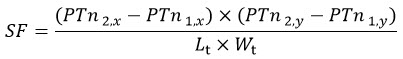Finding the shaded fraction could be implemented much more simply by using MATLAB’s rectangle intersection function Rectint, which requires as its arguments only the vertex vectors of polygons Sp and Pp.

10. For an entire array comprised of Nr rows or Nt columns (N in general) causing mutual shading, spaced equally, the shading fraction is slightly less because the first row or column remains unshaded, while the others are affected equally by their mutual shading.If multiple shadows from additional tables are considered, then μsh,B is calculated by a weighted average of the shading fraction on the relative number of tables.

For modeling portions of a larger array that has varying DC Field configurations, or when multiple small inverters and corresponding DC Fields make up an array, it may be preferred to assume that no unshaded columns- tables along the edge of the DC Field- exist. This is accomplished by assuming the Shading Factor applies to all tables in a row, regardless of proximity to the edge of the row, as though each row has an infinite number of tables. Proximity to the front of the array is still included, so the correct row count should be used. In order to consider the shading effects of surrounding DC fields and more accurately model shading losses for a DC Field as part of a larger array, this can be applied by activating Array-Based Shading in PlantPredict.

11. Reject all shading factors where the sun is found to be behind the plane of the array, i.e. the back of the module may be lit if the solar incidence angle is less than zero: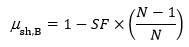The shading factor here is assigned to a value of -1 in order to distinguish it from 0 (fully shaded) and 1 (unshaded). A negative shading factor does not have any effect on the irradiance and will not contribute to the “shading loss” calculation.The shadows cast by surrounding tables are each determined independently based on their coordinate locations relative to the shaded table. Three cases exist: Transverse shading, which is caused by shading between tables across rows; Adjacent shading, which is caused by shading between tables within rows; and Diagonal shading, which is a combination of transverse and adjacent shading and is caused by tables located diagonally- neither within a row nor directly across rows. The formulas in the algorithm specific to each case are shown in the corresponding sections below identified by the relevant algorithm step.

The net shading fraction on the table is determined by evaluating the total area of the table covered by any of the shadows. This is calculated using an algorithm that determines the area affected by the shadows, accounting for the potential overlapping area of multiple shadows on the shared table.

The shadows considered in the current PlantPredict implementation are due to the tables shown in Figure 22 below, where each of the diagonal tables are in a dark or light red border.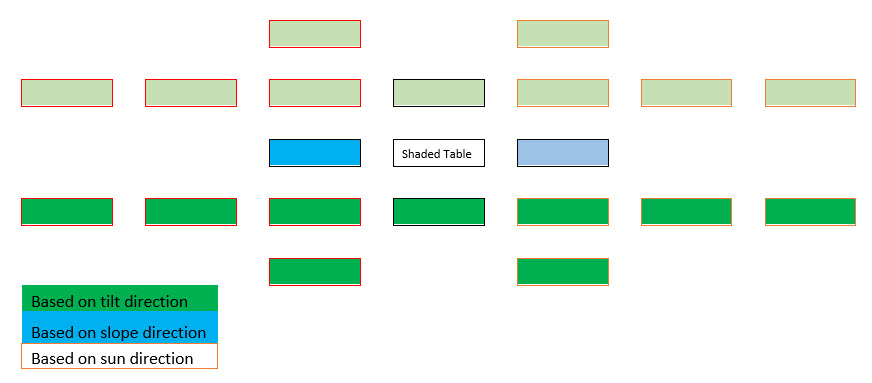### Figure 22. Tables from which shadows onto the shaded table are considered

Light green and dark green tables are considered depending on the tilt of the tables such that only the tables faced by the shaded table are considered. Light and dark blue tables are considered depending on the slope direction such that only the higher table is considered, since the lower table would never cast a shadow on front of the shaded table. Light and dark red-bordered tables are considered depending on the direction of the sun. These sets of tables are considered based on the sun azimuth angle relative to the azimuth angle faced by the tables.

To calculate the shadow, we need to know the vertical distance between the first row’s top edge and the second row’s top edge. This spacing is defined as the glass-to-glass height difference Δggrr and is a function of the post-to-post spacing Δpp and the slope of the ground in the row-to-row direction 𝜓.The tilt angle will always be positive for a fixed tilt system due to the user input restrictions. The primary row is always the one faced by the shaded row, which does not change for a fixed tilt system, but flips between rows (also known as columns) on either side of the shaded row, according to the tilt, for a tracker system. Therefore, for a tracker, the shadow of the table in the sun direction is considered based on the tilt by using the negative Δggrr for a negative tilt.

To calculate the shadow, we need to know the vertical distance between the first table’s top edge and the second table’s top edge, which can be calculated between any points along them since they are horizontal. This spacing is defined as the glass-to-glass height difference ΔggTT, and is a function of the table length LT, table-to-table spacing Lg, and the slope of the ground in the table-to-table direction Φ.Two adjustment factors, AdjFactor and bb1, are used to determine whether the primary table is in the positive or negative y-direction from the shaded table. This logic allows the algorithm to consider only the higher table along the row, which is the only one that can cast a shadow on the shaded table regardless of the sun direction.

For a tracker system,

AdjFactor = -1 and bb1 = 0, for slope Φ ≥ 0

For a fixed tilt system,

AdjFactor = -1 and bb1 = 0, for slope Φ < 0

Otherwise,

AdjFactor = 1 and bb1 = -1